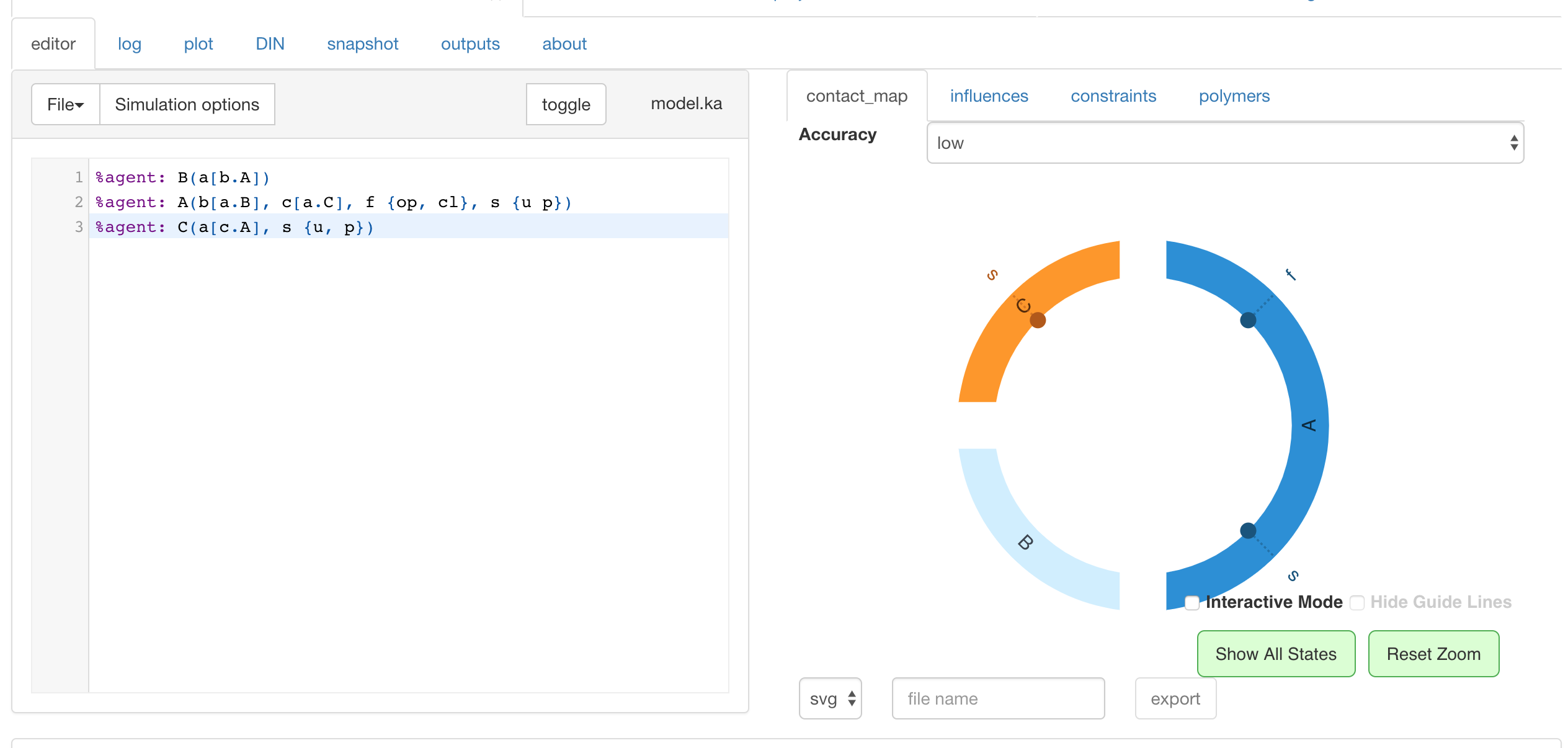# Protein interaction calculus

1. Recap:

• Versus:
• Molecular biology: understanding interactions (low level ≃ hardware)
• vs. Systems biology: understanding functions/behaviors (high level ≃ software engineering) ⟶ relation between activation of receptors, signaling cascades, and observed behavior (phenotype) (initially: to understand cancer)

• signaling pathways:

• Kinases: $A^{unphos} ⟶ A^{phos}$
• Phosphotases: $A^{phos} ⟶ A^{unphos}$
• One of the most studied pathways: Ras ⟶ Raf ⟶ MEK (induced by the growth factor): involved in cancer

• dotted arrow: postulated causality / headless arrows: inhibition
• Formal language (graph rewriting)
2. An ABC model

3. Stochastic simulation

A matter of time scale:

• Physics
• Chemistry
• Molecular biology (ns):
• protein folding (≃ ns)
• cell responses (≃ mn)/kappa
• Systems biology (≃ hr/day)

# Kappa

Kappa: we obviously don’t have confluence (not the same phenotypes) ⟹ we fix a reduction strategy.

In our case: stochastic strategy (non deterministically executed facts)

Example:

1. Kinase $B$ is a highly efficient enzyme that phosphorylates $A$

$A+B \leftrightarrow AB ⟶ A^\ast B \leftrightarrow A^\ast + B$
2. Kinase $A$ phosphorylates $C$
3. $A$ has a closed and open form
4. Kinase $B$ activates $A$

NB: ill-typed: the first 3 statements are at a molecular biology level, the last one pertains to systems biology (“activates”: it’s related to a function, so we don’t know how to interpret it: does it mean phosphorylation by $B$ or phosphorylation of $C$?)

cf. picture

in kappa:

%agent: B(a[b.A])
%agent: A(b[a.B], c[a.C], f {op, cl}, s {u p})
%agent: C(a[c.A], s {u, p}[.])


where

• [b.A]: constraint on links
• {p cl}: constraints on states
• [.]: constraint on links: it has to be freecf. pictures

Steady state of the system strongly biased toward “$A$ binds to $B$”: initially,

• there are

• $N_A × N_B$ patterns of the form $A, B$
• $0$ pattern of the form $A - B$
• after one binding, there are

• $(N_A-1) × (N_B-1)$ patterns of the form $A, B$
• $1$ pattern of the form $A - B$

⟹ strong biais towards creating bindings, as the rates are all the same

Product inhibition:

$A+B \leftrightarrow AB ⟶ A^\ast B \leftrightarrow A^\ast + B\\$

⟶ inefficient enzyme

Efficient enzyme:

$A+B \leftrightarrow AB ⟶ A^\ast B ⟶ A^\ast + B\\$

At home: try $A \text{ phosphorylated}$ ⟹ $A \text{ open}$, and then only $A \text{ open}$ phosphorylates $C$

## Stochastic strategies

Petri nets ≃ multi-set rewriting

For example:

$S, S, T ⟶ S, T, U$

But usually, written with

• Tokens: numbers of symbols $S, T, …$
• Thick lines: rules

In the literature:

• stochastic: probabilities not given, derived from the structure
• probabilistic: probabilities are given

Two things to compute:

1. which reaction should trigger? $ℙ_R, ℙ_S, …$
2. how long do I have to wait in order to see something happen? ⟹ compute the $dt$ increment

Monte-Carlo simulations: stochastic strategies developed to simulate these systems (invented for the Manhattan project):

• select two tokens at random and see if they can trigger a reaction

⟹ highly inefficient: times it takes to see when something happen depends on the CPU

but better: Gillespie’s algorithm (he’s a physicist) (1970’s) ⟶ introduced an algorithm that was standard in physics and became famous in the biology community

• what is the proba that $R$ triggers versus $S$?
• how long do you have to wait before a rule is triggered?

Which reaction should trigger?

Activity of the system:

$λ_{P_T} = \sum\limits_{ ρ ∈ \lbrace R, S \rbrace } \underbrace{λ_ρ}_{\text{activity of reaction } ρ}\\ λ_R = \vert P_A \vert × \vert P_B \vert × k_S\\ λ_S = \vert P_{AB} \vert × k_S\\ P_ρ = \frac{λ_ρ}{λ_{P_T}}$ $dt \sim λ_{P_T} \exp(-λ_{P_T} t) \sim \frac 1 {λ_{P_T}}$

Then: from Petri net to Continuous Time Markov Chain (CTMC)

cf. pictures

Tags:

Updated: